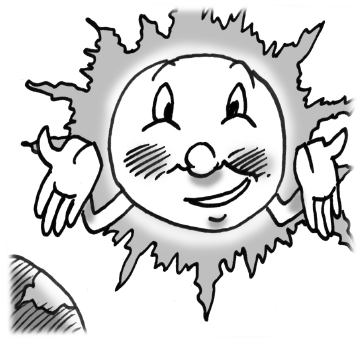### Home > INT2 > Chapter 12 > Lesson 12.1.4 > Problem12-51

12-51.Perhaps you think Earth is big? Consider the Sun!

1. Assume that the radius of Earth is $4000$ miles. The Sun is approximately $109$ times as wide. What is the Sun’s radius?

2. The distance between Earth and the Moon is $238{,}900$ miles. Compare this distance with the radius of the Sun you found in part (a).

3. If the Sun were hollow, how many Earths would fill the inside of it?

Width refers to the diameter of the earth.

$1{,}295{,}029$